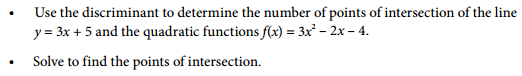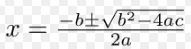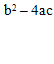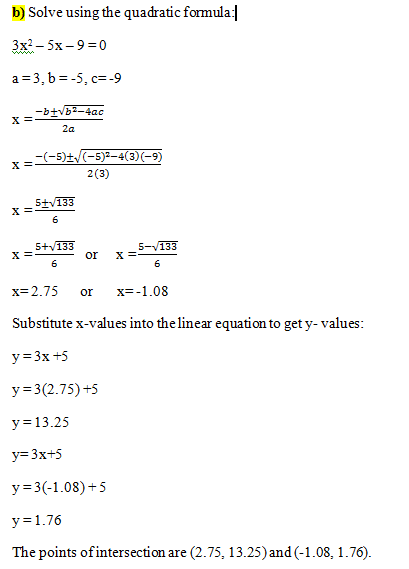# Use discriminant for linear and quad. function.

Evangeline101

## Homework Statement## Homework Equations## The Attempt at a Solution[/B]
Back again :) Is this correct?

Thanks.

#### Attachments

Mastermind01
Again you are correct.Mentor
2022 Award
Yes. Only that I have ##(-1.09 ; 1.73)## and ##(2.76 ; 13.27)##. But the path is correct.
It's always better to have exact numbers until the end and calculate the roots in a final step.

Homework Helper
Dearly Missed

## Homework Statement

View attachment 101055

## Homework Equations

View attachment 101057

View attachment 101061

## The Attempt at a Solution

View attachment 101062
View attachment 101063
[/B]
Back again :) Is this correct?

Thanks.

In thread after thread you ask "is this correct?...". That is a bad habit: as a matter of standard operating procedure you should check your answers for yourself (as you would need to do in an exam setting). Then, if you find something does not "work", you will know you have made an error somewhere, and asking for help then makes sense.

•Mastermind01
Mastermind01
In thread after thread you ask "is this correct?...". That is a bad habit: as a matter of standard operating procedure you should check your answers for yourself (as you would need to do in an exam setting). Then, if you find something does not "work", you will know you have made an error somewhere, and asking for help then makes sense.

I agree , I have already given the OP a way to check her work in a related similar thread.

Evangeline101
In thread after thread you ask "is this correct?...". That is a bad habit: as a matter of standard operating procedure you should check your answers for yourself (as you would need to do in an exam setting). Then, if you find something does not "work", you will know you have made an error somewhere, and asking for help then makes sense.

I understand, but can you suggest a less time consuming method than graphing, if I am doing an exam I won't have time to graph anything to check my answer. For this specific question, is there a way I can put the points back into the equation to verify, well maybe in this case I would have to graph since they are points of intersection.

Also I should be more clear when asking my question ( I will make sure to do that in future questions), in this case I would like to know if my process for part b is correct, does it make sense to use the linear equation to solve for y-values, and if so, is it because the linear equation is set equal to y?

Homework Helper
Dearly Missed
I understand, but can you suggest a less time consuming method than graphing, if I am doing an exam I won't have time to graph anything to check my answer. For this specific question, is there a way I can put the points back into the equation to verify, well maybe in this case I would have to graph since they are points of intersection.

Also I should be more clear when asking my question ( I will make sure to do that in future questions), in this case I would like to know if my process for part b is correct, does it make sense to use the linear equation to solve for y-values, and if so, is it because the linear equation is set equal to y?

To save time, don't graph anything; just check if your (x,y) values satisfy the requirement that they lie on both the line and the curve. That is easy: just plug in the numbers and calculate.

I have not checked your calculations for part (b), but I see that you have used the correct formulas. Again, you can check the solutions for yourself, by plugging in the numerical values and doing the calculations to see if everything works as it should.

Again: this is all just standard practice that you should get in the habit of doing.

Mentor
I understand, but can you suggest a less time consuming method than graphing, if I am doing an exam I won't have time to graph anything to check my answer.
Sure. You can substitute the points you found into each equation. If the point is on the graph, the equation should be a true statement.
In your work above, you found one solution to be ##x = \frac{5 + \sqrt{133}}{6}##. The corresponding y value is ##y = \frac{15 + \sqrt{133}}{2}## If you substitute this x value into the right sides of the equations ##y = 3x^2 - 2x - 4## and ##y = 3x + 5## you should get the y value I show above. Note that the values I show are the exact values. If you approximate the x values using a calculator, the y values you get will be only as precise as the rounding you do.
Evangeline101 said:
For this specific question, is there a way I can put the points back into the equation to verify, well maybe in this case I would have to graph since they are points of intersection.

Also I should be more clear when asking my question ( I will make sure to do that in future questions), in this case I would like to know if my process for part b is correct, does it make sense to use the linear equation to solve for y-values, and if so, is it because the linear equation is set equal to y?